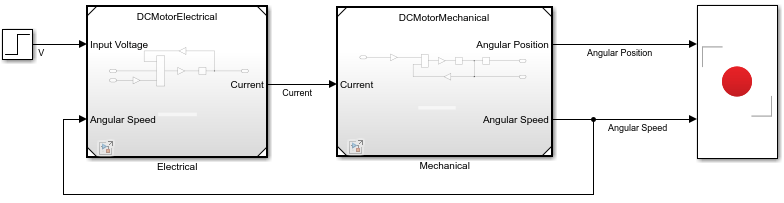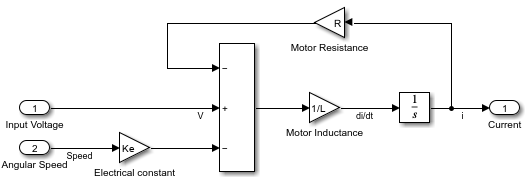# Use Model Reference with Local Solver in DC Motor

This example shows how to use local solvers to improve simulation performance in a DC motor with a mechanical load.

The subcomponents of a complex dynamic system often have different frequencies and time scales. For such systems, simulation speed can be accelerated by partitioning the model into different components and applying different solvers to components. Model reference with a local solver supports this approach by allowing the model reference with its own local solver to be referenced in a top model with a different solver.

### Analysis and Physics of the Model

In this DC motor model, torque is generated when an armature current flows through the armature coil. The torque is proportional to the armature current and the strength of the magnetic field of the motor. For a simple DC motor, you can assume that the magnetic field is constant and derive these equations .

Torque $T$ generated, where ${K}_{T}$ is the motor torque constant and $i$ is the armature current:

`$T={K}_{T}i$`

Electro magnetic field $e$ generated, where ${K}_{e}$ is the motor voltage constant and $Ɵ$ is the angle of the armature/load:

`$e={K}_{e}\frac{dƟ}{dt}$`

The DC motor is composed of two subcomponents:

• The electrical part, where $L$ is the inductance and$R$ is the resistance of the armature coil:

`$v=Ri+L\frac{di}{dt}+e=Ri+L\frac{di}{dt}+K\frac{dƟ}{dt}$`

• The mechanical part, where $J$ is the total moment of inertia of the motor armature plus load:

These two subcomponents are coupled through two states variables, current $i$ and the angular speed $\frac{dƟ}{dt}$.

The mechanical time constant can be approximated by assuming b = 0.

The electrical time constant is### Model Parameters and Time Constant

Assume these values for the parameters used in this example.

`$\begin{array}{ccc}\text{Parameter}& \text{Value}& \text{Unit}\\ {K}_{t}& 0.025& \text{Nm/A}\\ {K}_{e}& 0.025& \text{V/rad/s}\\ R& 3& \text{Ohm}\\ L& 2.5e-6& \text{H}\\ J& 3.2e-6& \text{Nm}{s}^{2}\\ b& 3.5e-6& \text{Nm/rad}\end{array}$`

Thus, s; and s.

If you use only one solver used to simulate this model, the step size required should be small enough to catch the electrical time constant. Assume a step size of ${t}_{\mathit{s}}=2\mathit{e}-7$.

The time constant of two subcomponents of the model is quite different. Thus, local solver of model reference can be used to accelerate the model.

### DC Model Using Model Reference

Based on the time constant analysis, you can partition the DC model into two components. Group the electrical part into a virtual subsystem so that it can be solved with the top solver. Group the mechanical part into a normal mode model reference so it can be solved with top solver or with the local solver. The DC motor is shown in this Simulink model.Partition the overall model into mechanical and electrical part and load the top model.

```topModel = 'DCMotor_localsolver'; bottomModel = 'DCMotor_Mechanical'; open_system(topModel) load_system(bottomModel)```

### Simulation

Use a global solver with the small step size of 2e-7 to simulate the model, then use this result as the baseline. Compute the time constants of the two components and use them to select the solver step size.

Calculate the electrical and mechanical system time constants `Tm` and `Te,` respectively. Calculate the time constant ratio that can be used to select the local solver step size. Also compute the global solver step based on time constant. Generally, you can calculate step size by dividing the time constant by 5.

```Tm = R*J/Ke/Kt; Te = L/R; timeConstantRatio = Tm/Te; topStep = 2E-7; localStep = topStep*10000; ```

Simulate the entire model with the top solver.

```set_param(topModel,'Solver','ode3','fixedStep', num2str(topStep)); set_param(bottomModel, 'UseModelRefSolver','off'); sim(topModel); y1 = yout.signals.values;```

Now, enable the local solver of the model reference that models the mechanical part of the model. Since the mechanical part has a much larger time constant then the electrical part, the step size of the local solver can be much larger than the global solver. Set the value to 2e-3.

Simulate the model with two solvers. For the top, use an ode`3` solver of step size 2e-7. For the local, use an `ode3` solver of step size 2e-2. When using a local solver, use zero-order hold (ZOH) data handling at the input and output of the model reference. The local solver parameters can be accessed through the solver tab of the Property Inspector of the model reference.

```set_param(topModel,'Solver','ode3','fixedStep',num2str(topStep)); set_param(bottomModel, 'UseModelRefSolver','on') set_param(bottomModel,'Solver','ode3','fixedstep',num2str(localStep)); ```

Set the instance-based parameters.

```mechanical = [topModel '/Mechanical']; set_param(mechanical,'InputSignalHandling','Zero-order hold'); set_param(mechanical,'OutputSignalHandling','Zero-order hold'); sim(topModel); y2 = yout.signals.values;```For Input Signal Handling, select 'auto' option. For Output Signal Handling, select 'use solver interpolant'. Finally, simulate the model with local fixed-step solver plus data exchange.

```set_param(topModel,'Solver','ode3','fixedStep',num2str(topStep)); set_param(bottomModel, 'UseModelRefSolver','on'); set_param(bottomModel,'Solver','ode3','fixedstep',num2str(localStep)); mechanical = [topModel '/Mechanical']; set_param(mechanical,'InputSignalHandling','Auto'); set_param(mechanical,'OutputSignalHandling','Use solver interpolant'); sim(topModel); sim(topModel); y3 = yout.signals.values;```

### Results

This plot compares three simulation results. The figure shows the position step response of the DC motor when voltage is applied to the terminal and the motor starts to rotate. The smooth blue curve is the baseline. The orange step curve shows the result of using local solver and ZOH input output data handling. This strategy samples the input and ouput of the mechanical component with a sample rate of 2e-3, and the local solver integrates its states with this step size. This strategy works well in this example as the result generated is fairly close to the baseline performance. The local solver using `'Auto'` and '`Use solver interpolant'` is the yellow curve. Compared to the ZOH strategy, this simulation result is even better. The orange line is smoother and closer to the baseline.

```figure(); plot(tout,y1,'LineWidth',2 );hold on; plot(tout,y2,'LineWidth',1.5);hold on; plot(tout,y3,'LineWidth',1.5);hold on; legend('global solver ode3', 'plus local solver ode3', 'plus data compensation'); title('Simualte DC Motor model with global and local solver'); xlabel('Time(s)'); ylabel('Position(rad)');```### Reference

 Younkin, George W. "Electric Servo Motor Equations and Time Constants." https://support.controltechnologycorp.com/customer/elearning/younkin/driveMotorEquations.pdf.